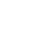Appendix E - Standard Built-in Filters

In this section the required RenderMan Interface filters are defined.  Keep in mind that the filter implementations may assume that they will never be passed (x,y) values that are outside the ([-xwidth/2, xwidth/2], [-ywidth/2,ywidth/2]) range.

Box Filter

RtFloat
RiBoxFilter (RtFloat x, RtFloat y, RtFloat xwidth, RtFloat ywidth)
{
return 1.0;
}

Triangle Filter

RtFloat
RiTriangleFilter (RtFloat x, RtFloat y, RtFloat xwidth, RtFloat ywidth)
{
return ( (1.0 - fabs(x)) / (xwidth*0.5) ) *
( (1.0 - fabs(y)) / (ywidth*0.5) );
}

CatmullRom Filter

RtFloat
RiCatmullRomFilter (RtFloat x, RtFloat y, RtFloat xwidth, RtFloat ywidth)
{
RtFloat r2 = (x*x + y*y);
RtFloat r = sqrt(r2);
return (r >= 2.0) ? 0.0 :
(r < 1.0) ? (3.0*r*r2 - 5.0*r2 + 2.0) :
(-r*r2 + 5.0*r2 - 8.0*r + 4.0);
}

Gaussian Filter

RtFloat
RiGaussianFilter (RtFloat x, RtFloat y, RtFloat xwidth, RtFloat ywidth)
{
x *= 2.0 / xwidth;
y *= 2.0 / ywidth;
return exp(-2.0 * (x*x + y*y));
}

Sinc Filter

RtFloat
RiSincFilter (RtFloat x, RtFloat y, RtFloat xwidth, RtFloat ywidth)
{
RtFloat s, t;
if (x > -0.001 && x < 0.001)
s = 1.0;
else
s = sin(x)/x;
if (y > -0.001 && y < 0.001)
t = 1.0;
else
t = sin(y)/y;
return s*t;
}No part of this publication may be reproduced, stored in a retrieval system, or transmitted, in any form or by any means, electronic, mechanical, photocopying, recording, or otherwise, without the prior written permission of Pixar. The information in this publication is furnished for informational use only, is subject to change without notice and should not be construed as a commitment by Pixar. Pixar assumes no responsibility or liability for any errors or inaccuracies that may appear in this publication.